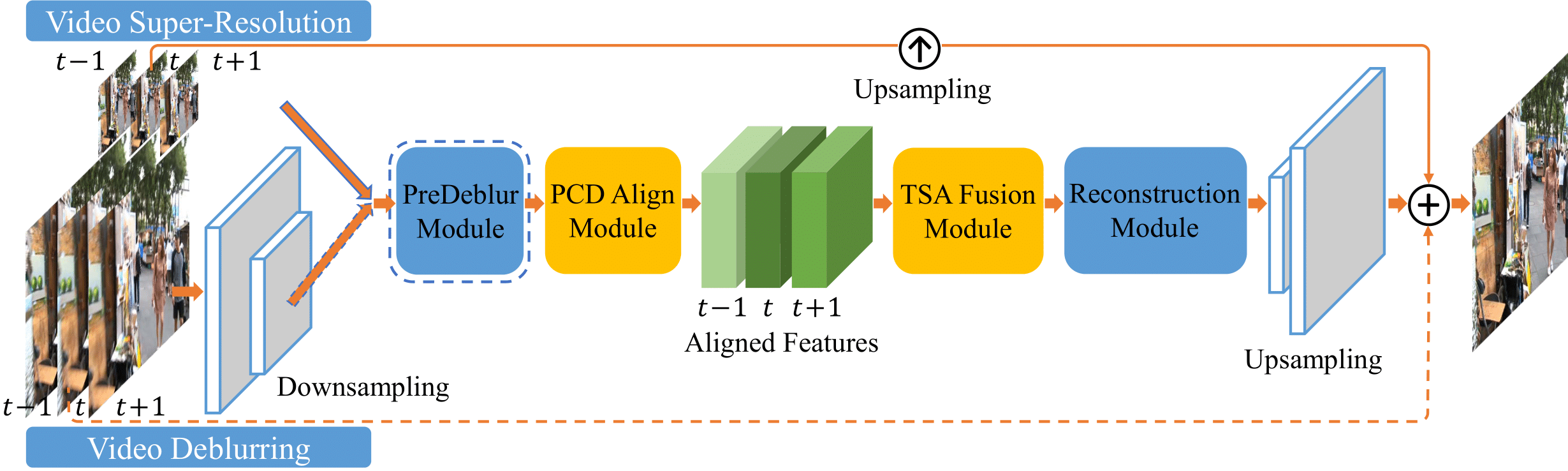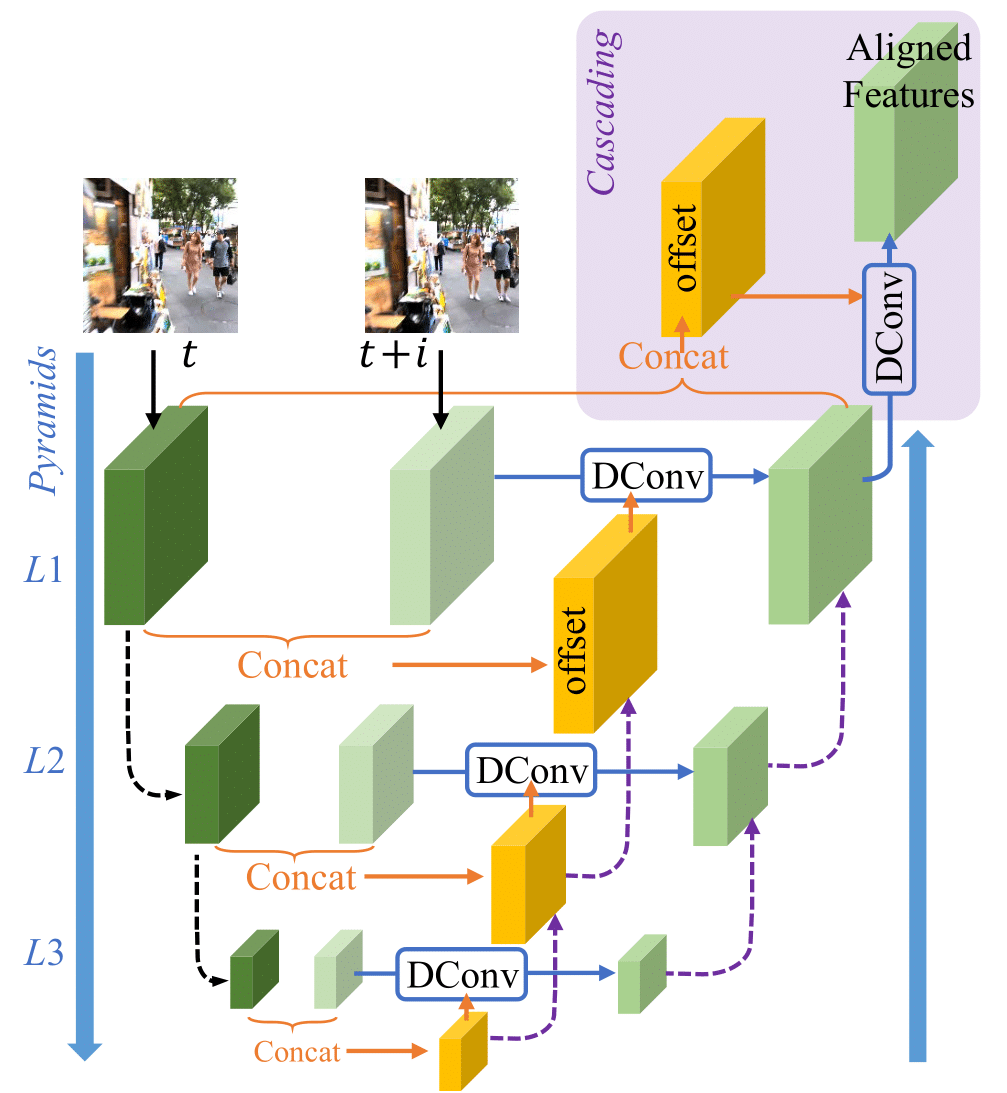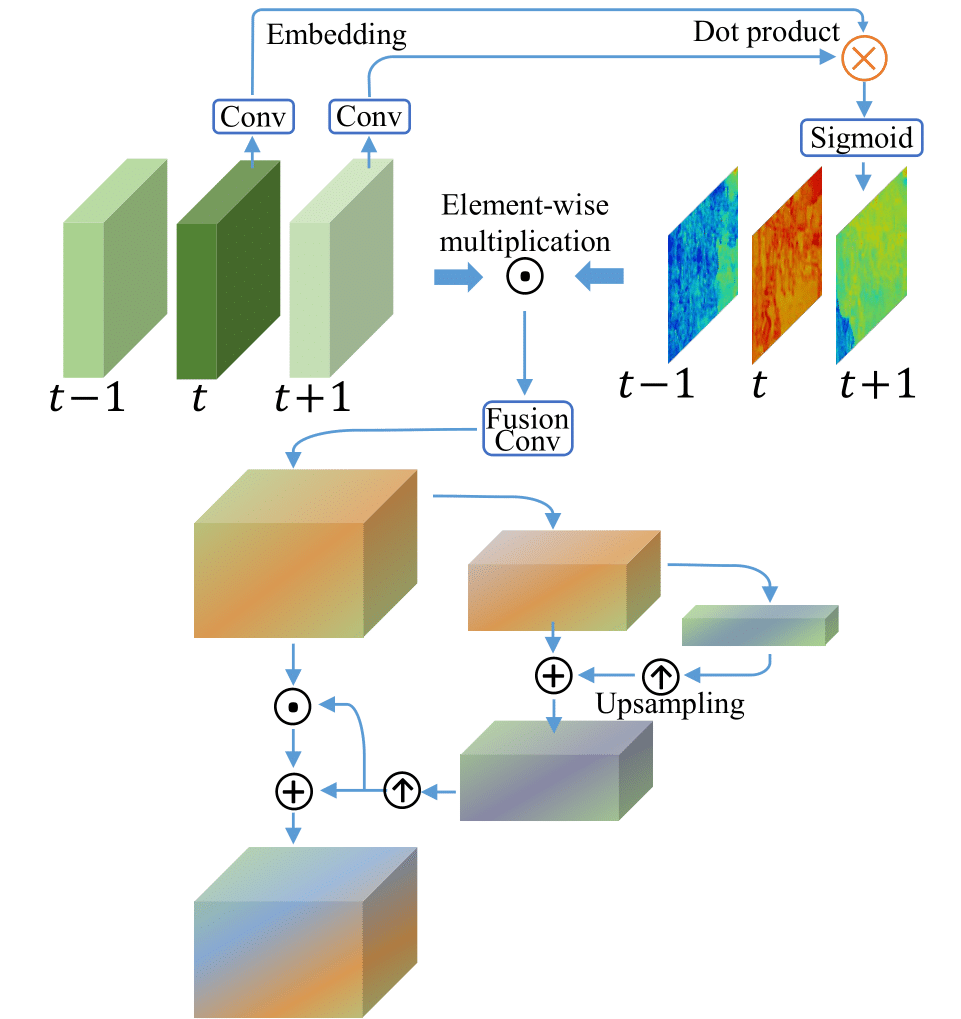# Overview

## The overall framework of EDVR

Given $2N+1$ consecutive frames $I_{[t-N:t+N]}$, denote middle frame $I_{t}$ as the reference frame and the other frames as neighboring framesInputs with high spatial resolution are first down-sampled to reduce computational cost. Given blurry inputs, a PreDeblur Module is inserted before the PCD Align Module to improve alignment accuracy. We use three input frames as an illustrative example.

• Downsampling & Upsampling:

If task is not Super Resolution with high spatial resolution inputs, input frames are first downsampled with strided convolution layers.

Resize the features back to the original input resolution in the upsampling layer at the end.

• PreDeblur:

This is used before the alignment module to pre-process blurry inputs and improve alignment accuracy.

• PCD:

Each neighboring frame is aligned to the reference one by the PCD alignment module at the feature level.

• TSA:

The TSA fusion module fuses image information of different frames.

• Reconstruction Module:

The fused features then pass through a reconstruction module, which is a cascade of residual blocks in EDVR and can be easily replaced by any other advanced modules in single image SR.

• Residual at the end:

High-resolution frame is obtained by adding the predicted image residual to a direct upsampled image (Super Resolution).

• Two-stage strategy:

Cascade the same EDVR network but with shallower depth to refine the output frames of the first stage.

The cascaded network can further remove the severe motion blur.

# Alignment with Pyramid, Cascading and Deformable Convolution (PCD)

## Use of Deformable Convolution

• Alignment features of each neighboring frame to reference frame.
• Different from optical-flow based method, deformable alignment is applied on the features of each frame, denote by $F_{t+i},i \in [{-}N{:}{+}N]$

• Learnable offset ($\Delta \mathbf{P}_{t+i}$) of Deformable Convolution is predicted as $f(\ [F_{t+i}, F_t]\ )$, where $f$ is general function consisting several convolution layers and $[F_{t+i}, F_t]$ is concat of two feature $F_{t+i}, F_t$.

## Pyramidal processing and Cascading refinement• Black dash lines:
• To generate feature F in l-th level, strided convolution filters are used to downsample the features at the (l-1)-th pyramid level by factor of 2.
• Orange lines:
• Concat reference frame with neighboring frame.
• The offset is made by convolution with concatenation.
• Purple dash lines:
• At the l-th level, offsets and aligned features are predicted also with X2 bilinear-interpolation-upsampled offsets and aligned features from the upper (l+1)-th level, respectively
• Output of Deformable Conv (blue line) and upsampled (l+1)-th level (purple dash lines) are mixed by general function with several convolution layers
• Light purple background:
• Following the pyramid structure, a subsequent deformable alignment is cascaded to further refine the coarsely aligned features.

# Fusion with Temporoal and Spatial Attention

Inter-frame temporal relation and intra-frame spatial relation are critical in fusion because

1. different neighboring frames are not equally informative due to occlusion, blurry regions and parallax problems
2. misalignment and unalignment arising from the preceding alignment stage adversely affect the subsequent reconstruction performance

→ Temporal and spatial attentions during the fusion process is adopted• Temporal Attention Maps: blue, red, green object of top left.

$F_{t}^{fusion} = \text{Conv}([\text{concat of attention-weighted features from t-N to t+N}])$